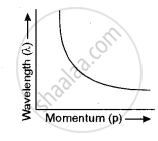# Show with the Help of a Labelled Graph How Their Wavelength (λ) Varies with Their Linear Momentum (P). - Physics (Theory)

Short Note

Show with the help of a labelled graph how their wavelength (λ) varies with their linear momentum (p).

#### Solution

The relation between λ and p isλ = h/p

Clearly, λ ∝ 1/p i.e.; p increases λ decreases as shown by the graph.

Concept: Wave Nature of Matter
Is there an error in this question or solution?